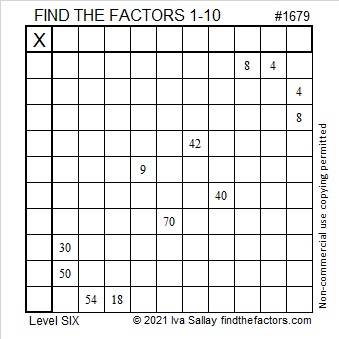# 1679 and Level 6

Contents

### Today’s Puzzle:

Can you complete this multiplication table puzzle? It’s a level 6, so some of the clues might be a little tricky. Use logic every step of the way, and everything will work out for you!### Factors of 1679:

• 1679 is a composite number.
• Prime factorization: 1679 = 23 × 73.
• 1679 has no exponents greater than 1 in its prime factorization, so √1679 cannot be simplified.
• The exponents in the prime factorization are 1 and 1. Adding one to each exponent and multiplying we get (1 + 1)(1 + 1) = 2 × 2 = 4. Therefore 1679 has exactly 4 factors.
• The factors of 1679 are outlined with their factor pair partners in the graphic below.### More About the Number 1679:

From OEIS.org we learn that
1 + 6 + 7 + 9 = 23, AND 1679 is divisible by 23.
This is cool: 1679 is the smallest multiple of 23 that can make that claim!

1679 is the hypotenuse of a Pythagorean triple:
1104-1265 -1679, which is 23 times (48-55-73).

This site uses Akismet to reduce spam. Learn how your comment data is processed.